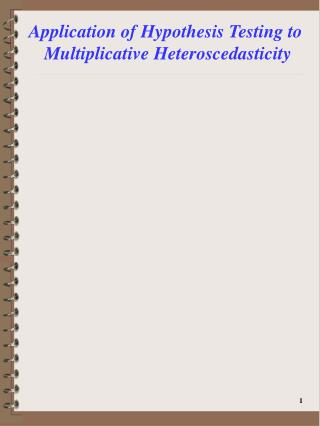# Application of Hypothesis Testing to Multiplicative Heteroscedasticity - PowerPoint PPT PresentationDownload PresentationApplication of Hypothesis Testing to Multiplicative Heteroscedasticity

Application of Hypothesis Testing to Multiplicative HeteroscedasticityDownload Presentation## Application of Hypothesis Testing to Multiplicative Heteroscedasticity

- - - - - - - - - - - - - - - - - - - - - - - - - - - E N D - - - - - - - - - - - - - - - - - - - - - - - - - - -
##### Presentation Transcript

1. Application of Hypothesis Testing to Multiplicative Heteroscedasticity

2. Application of Hypothesis Testing to Multiplicative Heteroscedasticity • Lets return to our general model where: • y=Xβ + e • y is a (T x 1) vector of obs. on the dependent variable • X is (T x K) matrix of obs. on the K exogenous variables • β is (K x 1) vector of parameters • e is a (T x 1) error vector where E(e)=0 and E(ee′) = Φ = σ2Ψ • Ψ is a (T x T) matrix assumed to depend on parameters Θ, Ψ = Ψ(Θ) • Dimension of Θ and how Ψ depends on Θ is a function of assumptions made about the data generating process (e.g., heteroscedasticy vs. autocorrelation) scaler

3. Application of Hypothesis Testing to Multiplicative Heteroscedasticity • If we assume that the error term and therefore y are both normally distributed, the total sample log likelihood function for (β,Θ) can be written as: Exogenous variables Φ(Θ*) = σ2Ψ(Θ) Θ* = σ2|Θ

4. Application of Hypothesis Testing to Multiplicative Heteroscedasticity Linear wrt β Nonlinear wrt α • Lets return to our multiplicative heteroscedasticy example we set up before where: • Yt=Xtβ + et E(e2t) = σ2t=exp(Wtα) =σ2exp(W*tα*) Wt=(1,W*t) t=(1,…,T) α' = (ln(σ2) α*) • Φ is a diagonal matrix w/tth diagonal element: σ2t=exp(Wtα) • JHGLL, p.538-541, 548-551, Greene, p.522-527

5. Application of Hypothesis Testing to Multiplicative Heteroscedasticity • If we assume the error terms follow a multivariate normal distribution then the sample log-likelihood is: σ2=exp(α1)

6. Application of Hypothesis Testing to Multiplicative Heteroscedasticity • Under this framework, the information matrix can be derived: S = # of variance exog. variables Assumed normal distribution For β’s W is TxS vector of exogenous data For α’s

7. Application of Hypothesis Testing to Multiplicative Heteroscedasticity ML Model of Multiplicative Hetero. Proc Defining Likelihood Function Dep. & Exog. Variables Numerical Gradients Maximum Likelihood Procedure Estimates of ,  Starting Values Proc for Calc. Variances , 

8. Application of Hypothesis Testing to Multiplicative Heteroscedasticity • ML estimation of multiplicative heteroscedasticity model • JHGLL, pg. 538-541, Table 9.1 • MATLAB Code uses the BHHH algorithm • I use the 2-step results as starting values for the ML estimation (K x 1) step BHHH Lt is tth contribution (K x K)

9. Application of Hypothesis Testing to Multiplicative Heteroscedasticity • ML estimation of multiplicative heteroscedasticity model • MATLAB Code: • ∂L/∂θ = Z, use numerical gradients • H = Z′Z Pn= -solve(H) • db = (solve(H)%*%colSums (z)) • Overview of Results Analytical Gradients LLF Function full step R commands H

10. Application of Hypothesis Testing to Multiplicative Heteroscedasticity • I use two methods to obtain parameter covariance matrix: • BHHH method (Numerical) • -Pn • Previous Analytical Analytical Gradient Function 10

11. Application of Hypothesis Testing to Multiplicative Heteroscedasticity • Testing for Multiplicative Heteroscedasticity Using 3 Asymptotic Tests • H0: Homoscedasticity H1: Multiplicative Heteroscedasticity • H0: α2=α3=…=αS=0 H1: at least one of the above ≠0 • Continuing with our example (Table 9.1 JHGLL)

12. Application of Hypothesis Testing to Multiplicative Heteroscedasticity • Wald Test • 1 element in α* • Remember Σα,ML=2(W′W)-1 • λW=(0.21732)2[(0.00379774)-1]/2 = 6.218 • χ21,.05=3.84→Reject H0 exog. variables est. coeff. (W*′W*)-1 Var(α2)=2*.003798=0.007596

13. Application of Hypothesis Testing to Multiplicative Heteroscedasticity • Lagrangian Multiplier Test • Information Matrix=1/2(W′W) • Σα= 2(W′W)-1 • Using the theoretical results shown in the previous handout λLM=4.028>3.84→reject H0 LM= S(0)′I(0)-1S(0)

14. Application of Hypothesis Testing to Multiplicative Heteroscedasticity • Likelihood Ratio Test • Using the full likelihood function LU=-61.748 LR=-64.315 λLR=2(-61.748-(-64.315))=5.134 difference in concentrated LLF’s χ21,.05=3.84

15. Application of Hypothesis Testing to Multiplicative Heteroscedasticity • Remember that in general: λW > λLR > λLM • In our empirical example: λW = 6.218 λLR = 5.204 λLM=4.028